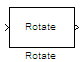# Rotate

Rotate image by specified angle

•Libraries:
Computer Vision Toolbox / Geometric Transformations

## Description

Use the Rotate block to rotate a grayscale or color image by an angle specified in radians.

## Ports

### Input

expand all

Image to rotate, specified as a 2-D numeric matrix for a grayscale image or 3-D numeric array for a color image.

Data Types: `single` | `double` | `int8` | `int16` | `int32` | `uint8` | `uint16` | `uint32` | `fixed point`

Rotation angle, specified as a numeric scalar, in radians.

If the input to the Image port is of data type `single`, `double`, or ```fixed point```, then the input to the Angle port must be of the same data type.

If the input to the Image port is of a signed or unsigned integer data type, then the input to the Angle port must be of a signed integer data type.

#### Dependencies

To enable this port, set the Rotation angle source parameter to `Input port`.

Data Types: `single` | `double` | `int8` | `int16` | `int32` | `fixed point`

### Output

expand all

Rotated image, returned as 2-D numeric matrix if the input image is a grayscale image and a 3-D numeric array if the input image is a color image.

Data Types: `single` | `double` | `int8` | `int16` | `int32` | `uint8` | `uint16` | `uint32` | `fixed point`

## Parameters

expand all

### Main

Specify the size of the rotated image as one of these options.

• `Expanded to fit rotated input image`- The block outputs a matrix or array expanded to contain all values.

• `Same as input image`- The block outputs a matrix or array the same size as the input image, which contains the middle part of the rotated image. This can result in the block cropping the edges of the rotated image.

Use the Background fill value parameter to specify the color or intensity value of pixels outside the rotated image.

Specify the source of the rotation angle as one of these options.

• `Specify via dialog` - Specify the rotation angle using the Angle (radians) parameter.

• `Input port` - Specify the rotation angle using the Angle port. The block uses the input to this port as the rotation angle at each time step.

Specify the rotation angle in radians.

When the rotation angle is a multiple of `pi/2`, the block uses a more efficient algorithm. If the specified value for the Angle (radians) parameter is within `1e-5` radians of a multiple of `pi/2`, the block rounds the angle value to that multiple of` pi/2` before performing the rotation.

#### Dependencies

To enable this parameter, set the Rotation angle source parameter to `Specify via dialog`.

Specify the maximum angle by which to rotate the input image as a scalar value in the range [`0`, `pi`], in radians. The block determines which angle, between `0` and the specified maximum angle, requires the largest output size and sets the dimensions of the output port accordingly.

#### Dependencies

To enable this parameter, specify these parameter values.

• Output size```Expanded to fit rotated input image```

• Rotation angle source```Input port```

Specify the position of the rotated image in the output image. If you select `Top-left corner`, the block positions the rotated image so that it has corners in contact with the top and left sides of the output image. If you select `Center`, the output image displays the rotated image at its center.

#### Dependencies

To enable this parameter, set the Rotation angle source parameter to `Input port`.

Specify the sine value computation method. If you select `Trigonometric function`, the block computes the sine and cosine values required to calculate the rotation of the image during the simulation.

If you select `Table lookup`, the block computes and stores the trigonometric values required to calculate the rotation of the image before the simulation starts. For this method, the block requires extra memory.

Specify a value for the pixels that are outside the image.

If the input image is grayscale, the value of Background fill value must be a numeric scalar. The default value is `0` for grayscale images. If the input image is a color image, the value of Background fill value must be a three-element numeric vector. The default value is `[0 0 0]` for color images.

Specify which interpolation method the block uses to rotate the image from these options.

• `Nearest neighbor` - The block uses the value of one nearby pixel for the new pixel value.

• `Bilinear` - The new pixel value is the weighted average of the 4 nearest pixel values.

• `Bicubic` - The new pixel value is the weighted average of the 16 nearest pixel values.

The number of pixels the block considers affects the complexity of the computation. Therefore, the `Nearest neighbor` interpolation is the most computationally efficient. However, because the accuracy of the method is proportional to the number of pixels considered, the `Bicubic` method is the most accurate.

### Data Types

For details on general fixed-point block parameters, see Specify Fixed-Point Attributes for Blocks.

Choose how to specify the word length and the fraction length of the angle values.

#### Dependencies

To enable this parameter, set the Rotation angle source parameter to `Specify via dialog`.

Select this parameter to prevent the fixed-point tools from overriding the data types you specify in this block. For more information, see Lock the Output Data Type Setting (Fixed-Point Designer).

## Block Characteristics

 Data Types `double` | `fixed point` | `integer` | `single` Multidimensional Signals `yes` Variable-Size Signals `yes`

## Algorithms

The Rotate block uses the three-pass shear rotation algorithm to compute its values, which differs from the algorithm used by the `imrotate` function in the Image Processing Toolbox™.

 Wolberg, George. Digital Image Warping. IEEE Computer Society Press Monograph. Los Alamitos, Calif: IEEE Computer Society Press, 1992.